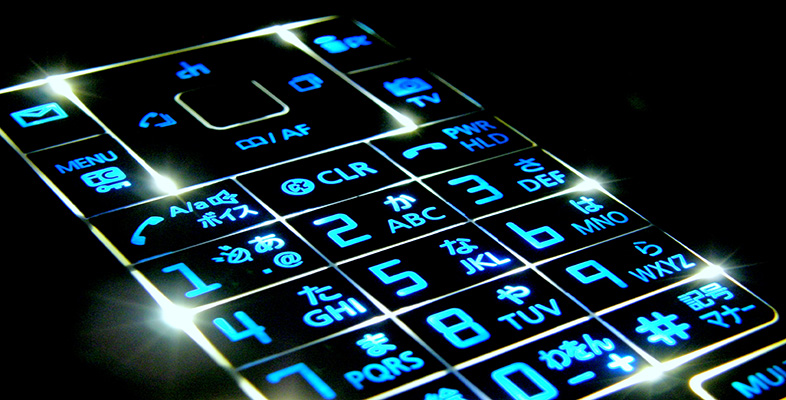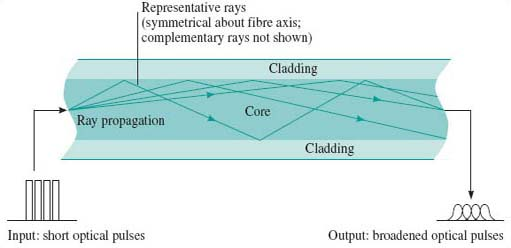Digital & Computing

### Become an OU studentDigital communications

Start this free course now. Just create an account and sign in. Enrol and complete the course for a free statement of participation or digital badge if available.

# 2.4.1 Multimode distortion

With multimode fibre, the main cause of pulses spreading is the multiple paths that signals can traverse as they travel along the fibre. This phenomenon of multimode distortion is illustrated in Figure 5.Figure 5 Multimode distortion

In the step-index fibre the light in the propagating modes is always in the core and therefore always propagating in glass with the same refractive index. The higher-order modes (the ones zig-zagging most) travel the furthest and therefore take the longest to propagate. In graded-index fibre the higher-order modes are in glass with a lower refractive index when they are off the axis. Light travels faster where the refractive index is lower, so in the graded-index fibre the light travels faster in the modes with the longer paths. This results, ideally, in the same overall propagation delay for all modes. A perfect balance is never possible, however, so there is still some pulse spreading due to multimode distortion even in graded-index fibre.

Spreading due to multimode distortion limits the usable bandwidth of a fibre. Since the amount of spreading is proportional to distance, the bandwidth is inversely proportional to distance, and the multimode distortion of a fibre is usually specified by the bandwidth-distance product.

Thus, for example, a 50/125 μm step-index fibre might have a bandwidth-distance product of 20 MHz·km. A 1 km fibre would have bandwidth of 20 MHz and a 2 km fibre would have a bandwidth of 10 MHz.

## Activity 5

There are two different 50 μm graded-index multimode fibres specified for use with Gigabit Ethernet. Used with a 850 nm source, they are specified with a bandwidth-distance product of 400 MHz·km and 500 MHz·km. (The earlier discussion of power budgets considered only the first of these two.)

Given that the signalling rate of Gigabit Ethernet is 1.25 Gbaud, what will be the maximum fibre length for each of these fibres before bandwidth becomes a problem?

The signalling rate, 1.25 Gbaud, is higher than the data rate, 1 GHz, because of the line coding. The line coding is 8B10B, which means that for every 8 bits there are 10 binary symbols, so the signalling rate is greater than the data rate by a factor 10/8.

Remember that to avoid inter-symbol interference the maximum signalling rate that can be transmitted through a channel of bandwidth B is the Nyquist rate of 2B.

The minimum bandwidth is half the signalling rate, which is 625 MHz. The maximum lengths that will give this bandwidth are:

(a) the maximum distance is 400/625 = 0.64 km, or 640 m

(b) the maximum distance is 500/625 = 0.8 km, or 800 m.

In practice the bandwidth is not a sharp cut-off, and there would be some degradation to the signal for distances shorter than this. In fact, the degradation caused by multimode distortion is one of the sources of the link power penalty used in the calculation of the power budget earlier. In the Gigabit Ethernet standard the link power penalty for the 400 MHz·km fibre is 4.1 dB, but only 3.6 dB for the 500 MHz·km fibre, and the operating distance specified for the 500 MHz·km fibre is 550 m compared to 500 m for the 400 MHz·km fibre.

## SAQ 5

1000BASE-SX over 62.5 μm multimode fibre is also specified for two different fibre bandwidth-distance products (Table 38-12 of IEEE 802.3):

• (a) 160 MHz·km

• (b) 200 MHz·km.

What will be the maximum fibre length before bandwidth becomes a problem with each of these fibres? (Remember that the signalling rate in Gigabit Ethernet is 1.25 Gbaud.)